Anúncio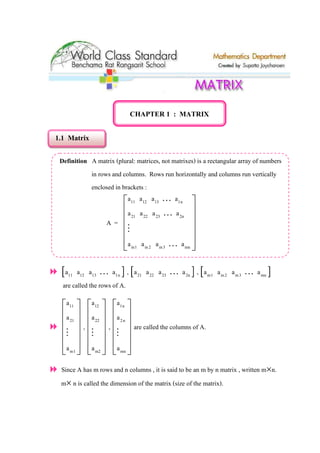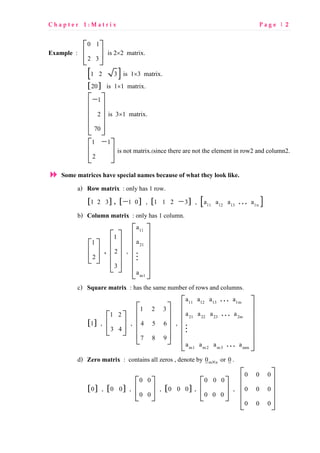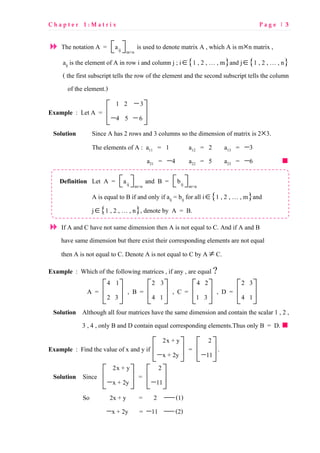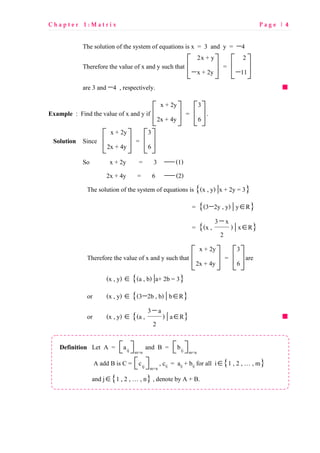Anúncio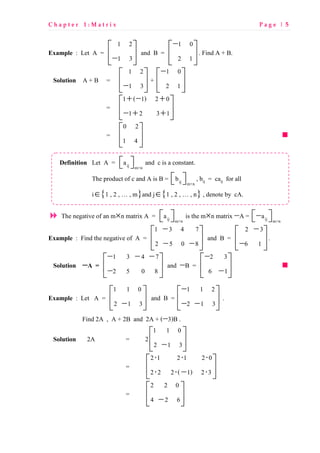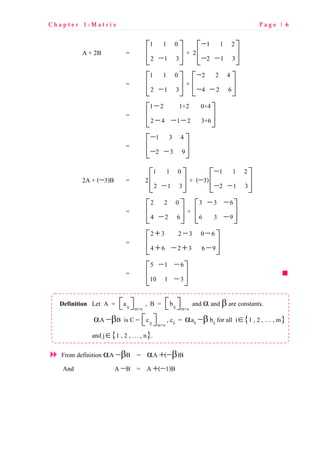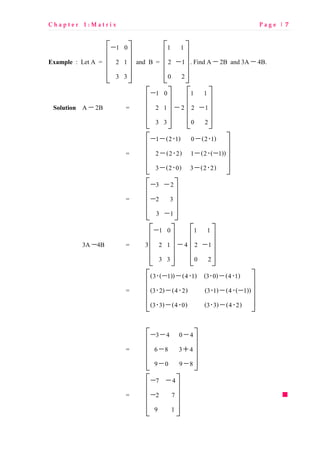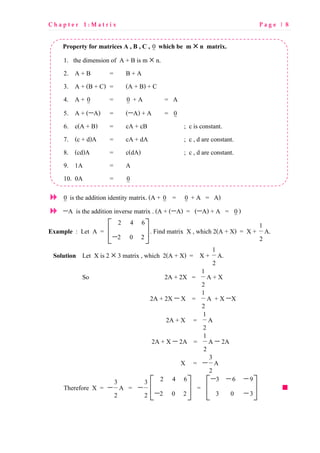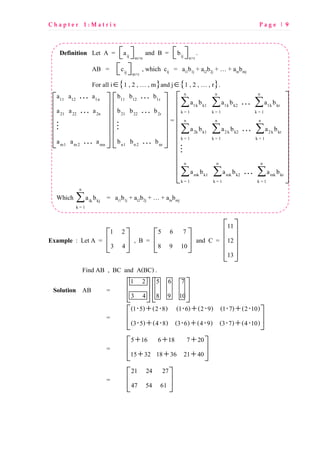Anúncio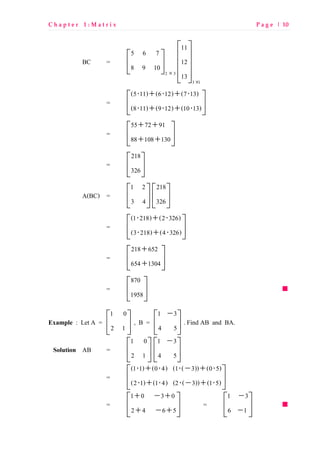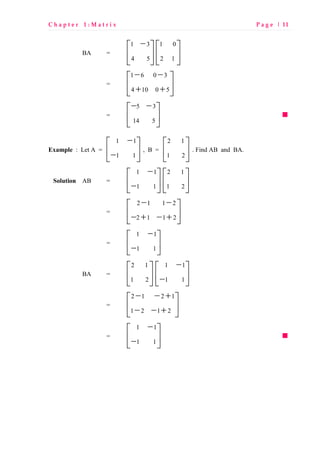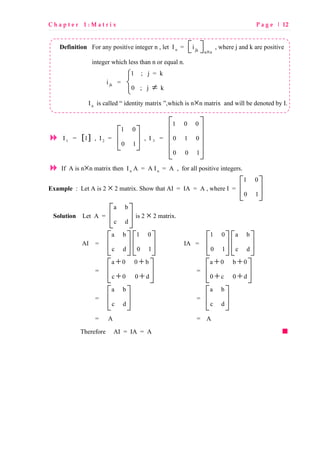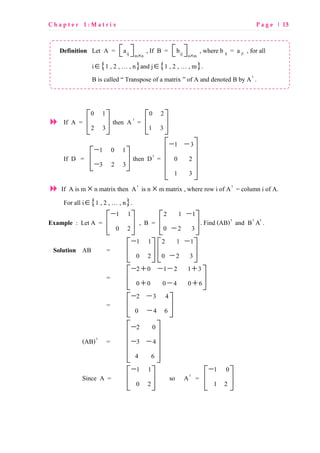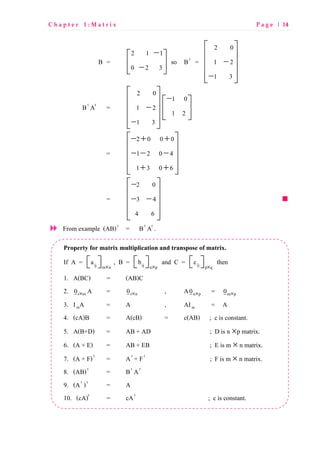Anúncio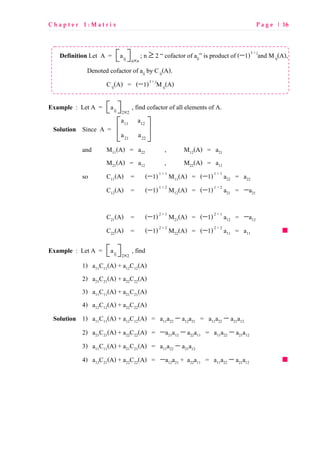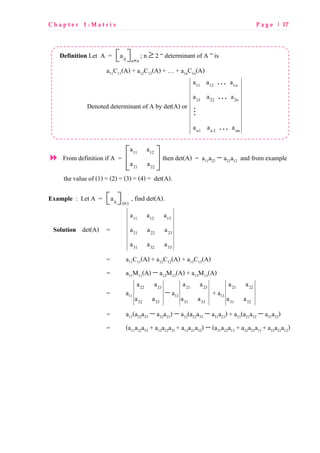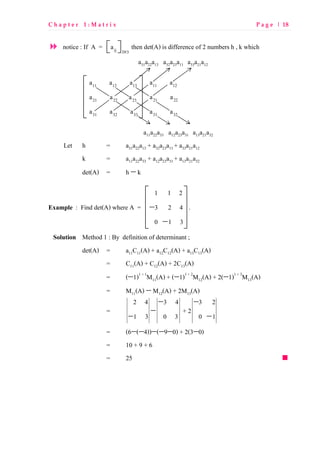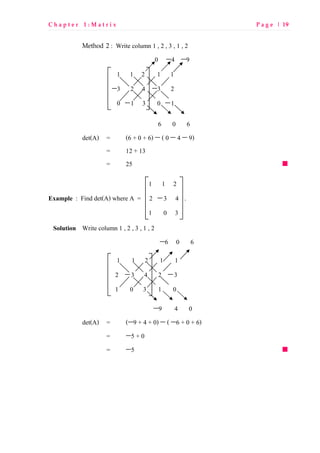Anúncio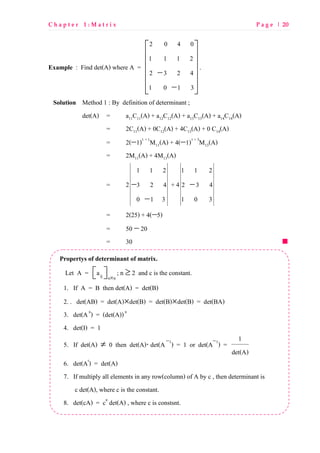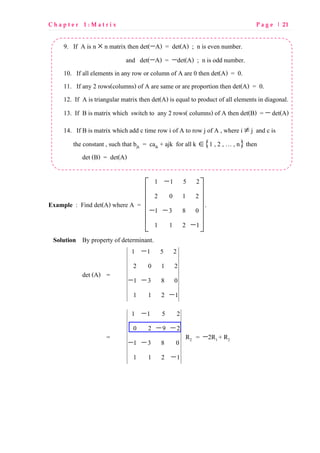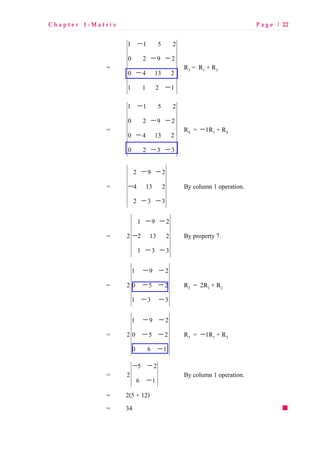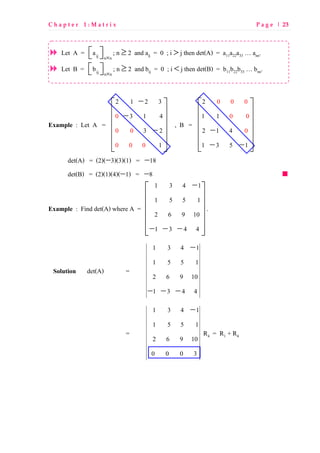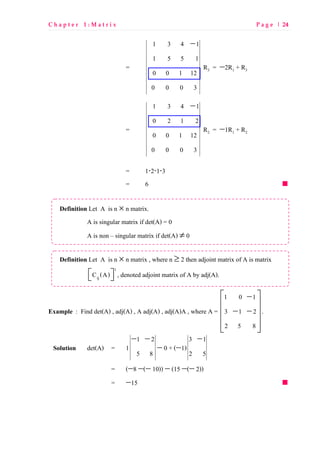Anúncio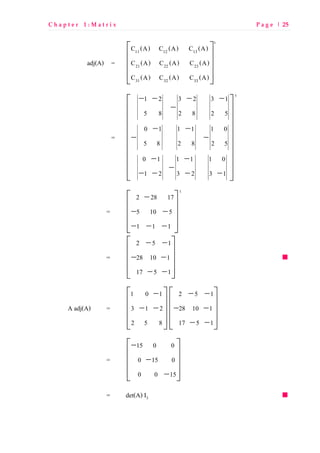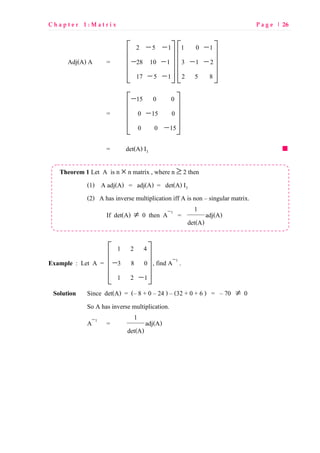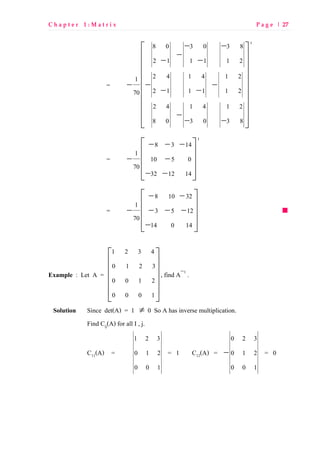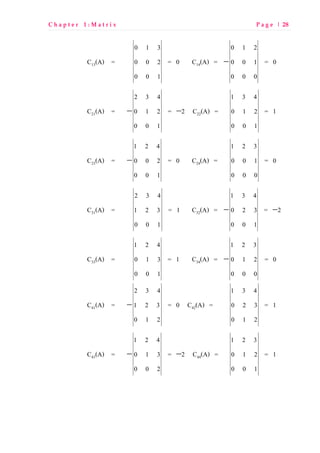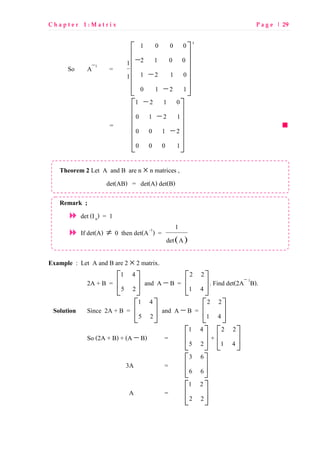Anúncio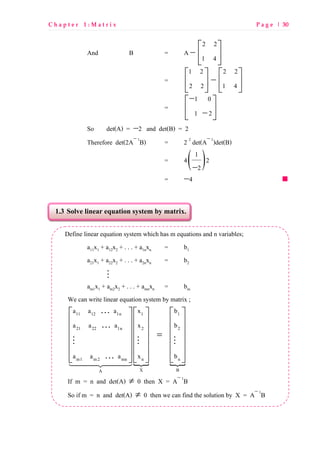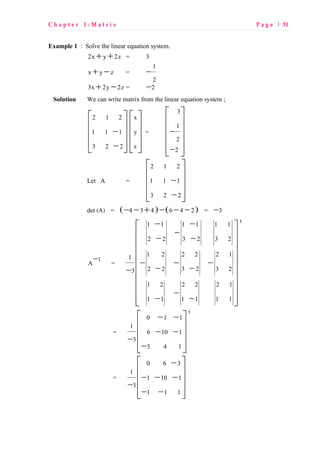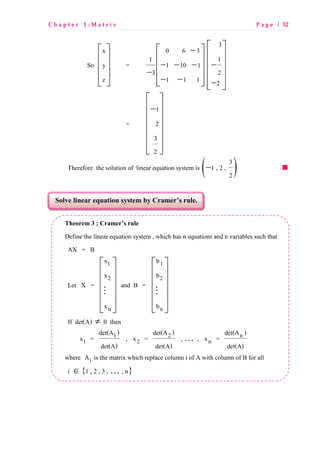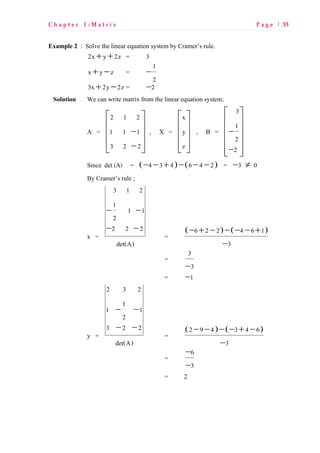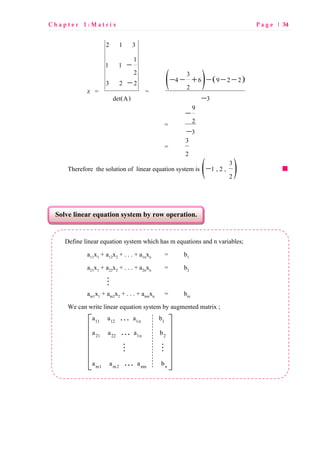Anúncio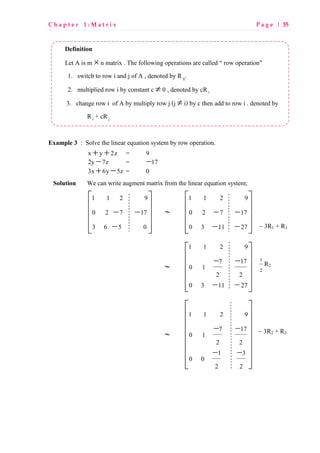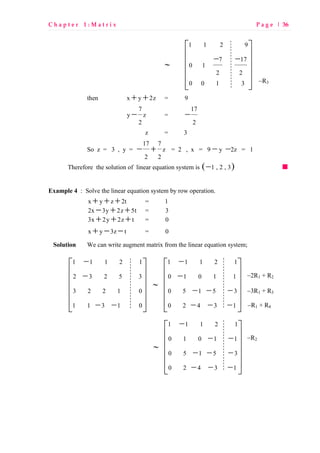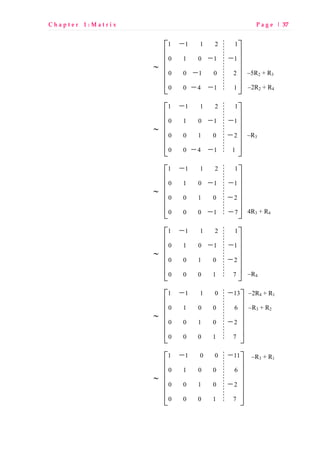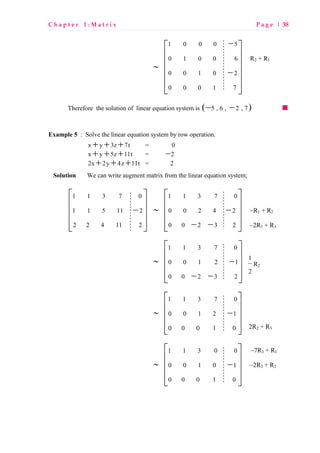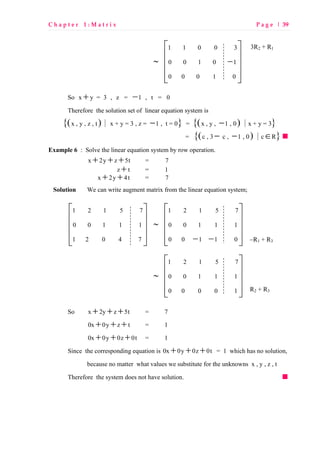Anúncio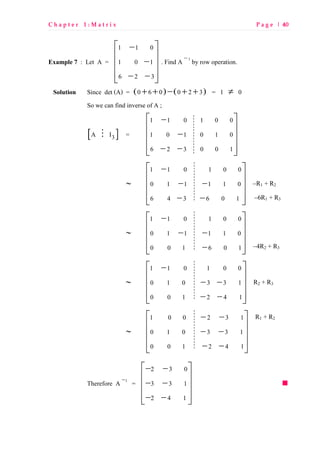Próximos SlideShares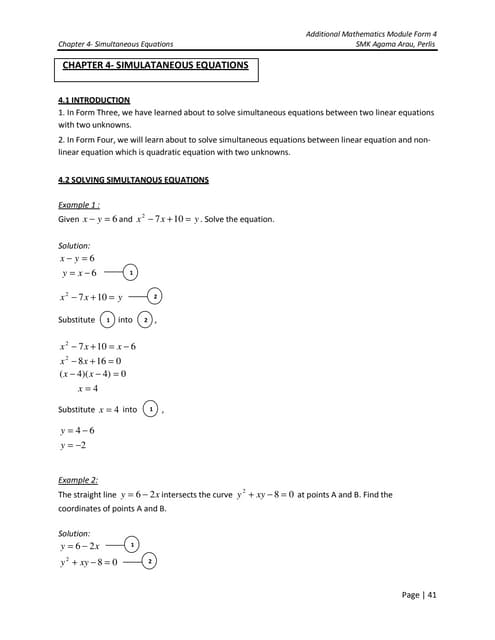Chapter 4 simultaneous equations
Carregando em ... 3
1 de 40
Anúncio

### Metrix

1. CHAPTER 1 : MATRIX 1.1 Matrix Definition A matrix (plural: matrices, not matrixes) is a rectangular array of numbers in rows and columns. Rows run horizontally and columns run vertically enclosed in brackets : A = 11 12 13 1n 21 22 23 2n m1 m2 m3 mn a a a a a a a a a a a a             K K M K [ ]11 12 13 1na a a aK , [ ]21 22 23 2na a a aK , [ ]m1 m2 m3 mna a a aK are called the rows of A. 11 21 m1 a a a             M , 12 22 m2 a a a             M , 1n 2n mn a a a             M are called the columns of A. Since A has m rows and n columns , it is said to be an m by n matrix , written m×n. m× n is called the dimension of the matrix (size of the matrix).
2. C h a p t e r 1 : M a t r i x P a g e | 2 Example : 0 1 2 3       is 2×2 matrix. [ ]1 2 3 is 1×3 matrix. [ ]20 is 1×1 matrix. 1 2 70  −         is 3×1 matrix. 1 1 2 −      is not matrix.(since there are not the element in row2 and column2. Some matrices have special names because of what they look like. a) Row matrix : only has 1 row. [ ]1 2 3 , [ ]1 0− , [ ]1 1 2 3− , [ ]11 12 13 1na a a aK b) Column matrix : only has 1 column. 1 2       , 1 2 3           , 11 21 m1 a a a             M c) Square matrix : has the same number of rows and columns. [ ]1 , 1 2 3 4       , 1 2 3 4 5 6 7 8 9           , 11 12 13 1m 21 22 23 2m m1 m2 m3 mm a a a a a a a a a a a a             K K M K d) Zero matrix : contains all zeros , denote by m n0 × or 0. [ ]0 , [ ]0 0 , 0 0 0 0       , [ ]0 0 0 , 0 0 0 0 0 0       , 0 0 0 0 0 0 0 0 0          
3. C h a p t e r 1 : M a t r i x P a g e | 3 The notation A = ij m×n a   is used to denote matrix A , which A is m×n matrix , aij is the element of A in row i and column j ; i∈{1 , 2 , 1 , m}and j∈{1 , 2 , 1 , n} ( the first subscript tells the row of the element and the second subscript tells the column of the element.) Example : Let A = 1 2 3 4 5 6 −   − −  Solution Since A has 2 rows and 3 columns so the dimension of matrix is 2×3. The elements of A : a11 = 1 a12 = 2 a13 = −3 a21 = −4 a22 = 5 a23 = −6 Definition Let A = ij m×n a   and B = ij m×n b   A is equal to B if and only if aij = bij for all i∈{1 , 2 , 1 , m}and j∈{1 , 2 , 1 , n}, denote by A = B. If A and C have not same dimension then A is not equal to C. And if A and B have same dimension but there exist their corresponding elements are not equal then A is not equal to C. Denote A is not equal to C by A ≠ C. Example : Which of the following matrices , if any , are equal ? A = 4 1 2 3       , B = 2 3 4 1       , C = 4 2 1 3       , D = 2 3 4 1       Solution Although all four matrices have the same dimension and contain the scalar 1 , 2 , 3 , 4 , only B and D contain equal corresponding elements.Thus only B = D. Example : Find the value of x and y if 2x + y 2 = x + 2y 11        − −    . Solution Since 2x + y 2 = x + 2y 11        − −    So 2x + y = 2  (1) −x + 2y = −11  (2)
4. C h a p t e r 1 : M a t r i x P a g e | 4 The solution of the system of equations is x = 3 and y = −4 Therefore the value of x and y such that 2x + y 2 = x + 2y 11        − −    are 3 and −4 , respectively. Example : Find the value of x and y if x + 2y 3 = 2x + 4y 6             . Solution Since x + 2y 3 = 2x + 4y 6             So x + 2y = 3  (1) 2x + 4y = 6  (2) The solution of the system of equations is {(x , y) |x + 2y = 3} = {(3−2y , y) | y∈R} = {(x , 3 x 2 − ) | x∈R} Therefore the value of x and y such that x + 2y 3 = 2x + 4y 6             are (x , y) ∈ {(a , b) |a+ 2b = 3} or (x , y) ∈ {(3−2b , b) | b∈R} or (x , y) ∈ {(a , 3 a 2 − ) | a∈R} Definition Let A = ij m×n a   and B = ij m×n b   A add B is C = ij m×n c   , cij = aij + bij for all i∈{1 , 2 , 1 , m} and j∈{1 , 2 , 1 , n} , denote by A + B.
5. C h a p t e r 1 : M a t r i x P a g e | 5 Example : Let A = 1 2 1 3    −  and B = 1 0 2 1 −      . Find A + B. Solution A + B = 1 2 1 3    −  + 1 0 2 1 −      = 1 ( 1) 2 0 1 2 3 1 + − +   − + +  = 0 2 1 4       Definition Let A = ij m×n a   and c is a constant. The product of c and A is B = ij m×n b   , bij = caij for all i∈{1 , 2 , 1 , m}and j∈{1 , 2 , 1 , n} , denote by cA. The negative of an m×n matrix A = ij m×n a   is the m×n matrix −A = ij m×n a−   Example : Find the negative of A = 1 3 4 7 2 5 0 8 −   − −  and B = 2 3 6 1 −   −  . Solution −−−−A = 1 3 4 7 2 5 0 8 − − −   −  and −B = 2 3 6 1 −   −  Example : Let A = 1 1 0 2 1 3    −  and B = 1 1 2 2 1 3 −   − −  . Find 2A , A + 2B and 2A + (−3)B . Solution 2A = 2 1 1 0 2 1 3    −  = 2 1 2 1 2 0 2 2 2 ( 1) 2 3 ⋅ ⋅ ⋅   ⋅ ⋅ − ⋅  = 2 2 0 4 2 6    − 
6. C h a p t e r 1 : M a t r i x P a g e | 6 A + 2B = 1 1 0 2 1 3    −  + 2 1 1 2 2 1 3 −   − −  = 1 1 0 2 1 3    −  + 2 2 4 4 2 6 −   − −  = 1 2 1+2 0+4 2 4 1 2 3+6 −   − − −  = 1 3 4 2 3 9 −   − −  2A + (−3)B = 2 1 1 0 2 1 3    −  + (−3) 1 1 2 2 1 3 −   − −  = 2 2 0 4 2 6    −  + 3 3 6 6 3 9 − −   −  = 2 3 2 3 0 6 4 6 2 3 6 9 + − −   + − + −  = 5 1 6 10 1 3 − −   −  Definition Let A = ij m×n a   , B = ij m×n b   and α and β are constants. αA −βB is C = ij m×n c   , cij = αaij −β bij for all i∈{1 , 2 , 1 , m} and j∈{1 , 2 , 1 , n}. From definition αA −βB = αA +(−β)B And A −B = A +(−1)B
7. C h a p t e r 1 : M a t r i x P a g e | 7 Example : Let A = 1 0 2 1 3 3  −         and B = 1 1 2 1 0 2     −      . Find A − 2B and 3A − 4B. Solution A − 2B = 1 0 2 1 3 3  −         − 2 1 1 2 1 0 2     −      = 1 (2 1) 0 (2 1) 2 (2 2) 1 (2 ( 1)) 3 (2 0) 3 (2 2)  − − ⋅ − ⋅   − ⋅ − ⋅ −   − ⋅ − ⋅  = 3 2 2 3 3 1  − −   −   −  3A −4B = 3 1 0 2 1 3 3  −         − 4 1 1 2 1 0 2     −      = (3 ( 1)) (4 1) (3 0) (4 1) (3 2) (4 2) (3 1) (4 ( 1)) (3 3) (4 0) (3 3) (4 2)  ⋅ − − ⋅ ⋅ − ⋅   ⋅ − ⋅ ⋅ − ⋅ −   ⋅ − ⋅ ⋅ − ⋅  = 3 4 0 4 6 8 3 4 9 0 9 8  − − −   − +   − −  = 7 4 2 7 9 1  − −   −     
8. C h a p t e r 1 : M a t r i x P a g e | 8 Property for matrices A , B , C , 0 which be m ×××× n matrix. 1. the dimension of A + B is m × n. 2. A + B = B + A 3. A + (B + C) = (A + B) + C 4. A + 0 = 0 + A = A 5. A + (−A) = (−A) + A = 0 6. c(A + B) = cA + cB ; c is constant. 7. (c + d)A = cA + dA ; c , d are constant. 8. (cd)A = c(dA) ; c , d are constant. 9. 1A = A 10. 0A = 0 0 is the addition identity matrix. (A + 0 = 0 + A = A) −A is the addition inverse matrix . (A + (−A) = (−A) + A = 0) Example : Let A = 2 4 6 2 0 2    −  . Find matrix X , which 2(A + X) = X + 1 2 A. Solution Let X is 2 × 3 matrix , which 2(A + X) = X + 1 2 A. So 2A + 2X = 1 2 A + X 2A + 2X − X = 1 2 A + X −X 2A + X = 1 2 A 2A + X − 2A = 1 2 A − 2A X = 3 2 − A Therefore X = 3 2 − A = 3 2 − 2 4 6 2 0 2    −  = 3 6 9 3 0 3 − − −   − 
9. C h a p t e r 1 : M a t r i x P a g e | 9 Definition Let A = ij m×n a   and B = ij n×r b   . AB = ij m×r c   , which cij = ai1b1j + ai2b2j + 1 + ainbmj For all i∈{1 , 2 , 1 , m}and j∈{1 , 2 , 1 , r}. 11 12 1n 21 22 2n m1 m2 mn a a a a a a a a a             K K M K 11 12 1r 21 22 2r n1 n2 nr b b b b b b b b b             K K M K = Which n ik kj k = 1 a b∑ = ai1b1j + ai2b2j + 1 + ainbmj Example : Let A = 1 2 3 4       , B = 5 6 7 8 9 10       and C = 11 12 13           Find AB , BC and A(BC) . Solution AB = 1 2 3 4       5 6 7 8 9 10       = (1 5) (2 8) (1 6) (2 9) (1 7) (2 10) (3 5) (4 8) (3 6) (4 9) (3 7) (4 10) ⋅ + ⋅ ⋅ + ⋅ ⋅ + ⋅   ⋅ + ⋅ ⋅ + ⋅ ⋅ + ⋅  = 5 16 6 18 7 20 15 32 18 36 21 40 + + +   + + +  = 21 24 27 47 54 61       n n n 1k k1 1k k2 1k kr k = 1 k = 1 k = 1 n n n 2k k1 2k k2 2k kr k = 1 k = 1 k = 1 n n n mk k1 mk k2 mk kr k = 1 k = 1 k = 1 a b a b a b a b a b a b a b a b a b                     ∑ ∑ ∑ ∑ ∑ ∑ ∑ ∑ ∑ K K M K
10. C h a p t e r 1 : M a t r i x P a g e | 10 BC = 2 3 5 6 7 8 9 10 ×       3 1 11 12 13 ×           = (5 11) (6 12) (7 13) (8 11) (9 12) (10 13) ⋅ + ⋅ + ⋅   ⋅ + ⋅ + ⋅  = 55 72 91 88 108 130 + +   + +  = 218 326       A(BC) = 1 2 3 4       218 326       = (1 218) (2 326) (3 218) (4 326) ⋅ + ⋅   ⋅ + ⋅  = 218 652 654 1304 +   +  = 870 1958       Example : Let A = 1 0 2 1       , B = 1 3 4 5 −      . Find AB and BA. Solution AB = 1 0 2 1       1 3 4 5 −      = (1 1) (0 4) (1 ( 3)) (0 5) (2 1) (1 4) (2 ( 3)) (1 5) ⋅ + ⋅ ⋅ − + ⋅   ⋅ + ⋅ ⋅ − + ⋅  = 1 0 3 0 2 4 6 5 + − +   + − +  = 1 3 6 1 −   − 
11. C h a p t e r 1 : M a t r i x P a g e | 11 BA = 1 3 4 5 −      1 0 2 1       = 1 6 0 3 4 10 0 5 − −   + +  = 5 3 14 5 − −      Example : Let A = 1 1 1 1 −   −  , B = 2 1 1 2       . Find AB and BA. Solution AB = 1 1 1 1 −   −  2 1 1 2       = 2 1 1 2 2 1 1 2 − −   − + − +  = 1 1 1 1 −   −  BA = 2 1 1 2       1 1 1 1 −   −  = 2 1 2 1 1 2 1 2 − − +   − − +  = 1 1 1 1 −   − 
12. C h a p t e r 1 : M a t r i x P a g e | 12 Definition For any positive integer n , let In = jk n n i ×    , where j and k are positive integer which less than n or equal n. ijk = 1 ; j = k 0 ; j k   ≠ In is called < identity matrix =,which is n×n matrix and will be denoted by I. I1 = [ ]1 , I2 = 1 0 0 1       , I 3 = 1 0 0 0 1 0 0 0 1           If A is n×n matrix then In A = A In = A , for all positive integers. Example : Let A is 2 × 2 matrix. Show that AI = IA = A , where I = 1 0 0 1       Solution Let A = a b c d       is 2 × 2 matrix. AI = a b c d       1 0 0 1       IA = 1 0 0 1       a b c d       = a 0 0 b c 0 0 d + +   + +  = a 0 b 0 0 c 0 d + +   + +  = a b c d       = a b c d       = A = A Therefore AI = IA = A
13. C h a p t e r 1 : M a t r i x P a g e | 13 Definition Let A = ij m n a ×    , If B = ij n m b ×    , where b ij = a ji , for all i∈{1 , 2 , 1 , n}and j∈{1 , 2 , 1 , m}. B is called < Transpose of a matrix = of A and denoted B by At . If A = 0 1 2 3       then A t = 0 2 1 3       If D = 1 0 1 3 2 3 −   −  then Dt = 1 3 0 2 1 3  − −         If A is m × n matrix then At is n × m matrix , where row i of At = column i of A. For all i∈{1 , 2 , 1 , n}. Example : Let A = 1 1 0 2 −      , B = 2 1 1 0 2 3 −   −  . Find (AB)t and Bt At . Solution AB = 1 1 0 2 −      2 1 1 0 2 3 −   −  = 2 0 1 2 1 3 0 0 0 4 0 6 − + − − +   + − +  = 2 3 4 0 4 6 − −   −  (AB)t = 2 0 3 4 4 6  −   − −      Since A = 1 1 0 2 −      so At = 1 0 1 2 −     
14. C h a p t e r 1 : M a t r i x P a g e | 14 B = 2 1 1 0 2 3 −   −  so Bt = 2 0 1 2 1 3     −   −  Bt At = 2 0 1 2 1 3     −   −  1 0 1 2 −      = 2 0 0 0 1 2 0 4 1 3 0 6  − + +   − − −   + +  = 2 0 3 4 4 6  −   − −      From example (AB)t = Bt At . Property for matrix multiplication and transpose of matrix. If A = ij m n a ×    , B = ij n p b ×    and C = ij p q c ×    then 1. A(BC) = (AB)C 2. r m0 × A = r n0 × , A n p0 × = m p0 × 3. ImA = A , AIm = A 4. (cA)B = A(cB) = c(AB) ; c is constant. 5. A(B+D) = AB + AD ; D is n ×p matrix. 6. (A + E) = AB + EB ; E is m × n matrix. 7. (A + F)t = At + Ft ; F is m × n matrix. 8. (AB)t = Bt At 9. (At )t = A 10. (cA)t = cAt ; c is constant.
15. C h a p t e r 1 : M a t r i x P a g e | 15 1.2 Inverse Matrix Definition Let A is n × n matrix if B is n × n matrix which AB = BA = In then B is called < inverse of a matrix = of A and denoted B by A−1 . If A = a b c d       and ad ? bc ≠ 0 then A has inverse and A −1 = d b1 c aad bc −   −−   Example : Find A −1 , where A = 2 2 4 1 −      . Solution Since (2×1) ? (4×(−2)) = 10 ≠ 0 Therefore A −1 = 1 21 4 210    −  Definition Let A = ij 1 1 a ×    , a is called < determinant = of A . Definition Let A = ij n n a ×    ; n ≥ 2 < minor of aij= is determinant of matrix which removed row i and column j from A , Denoted minor of aij by Mij(A). Example : Let A = ij 2 2 a ×    , find minor of all elements of A. Solution Since A = 11 12 21 22 a a a a       So M11(A) = a22 , M12(A) = a21 M21(A) = a12 , M22(A) = a11
16. C h a p t e r 1 : M a t r i x P a g e | 16 Definition Let A = ij n n a ×    ; n ≥ 2 < cofactor of aij= is product of (−1)I + j and Mij(A), Denoted cofactor of aij by Cij(A). Cij(A) = (−1)I + j Mij(A) Example : Let A = ij 2 2 a ×    , find cofactor of all elements of A. Solution Since A = 11 12 21 22 a a a a       and M11(A) = a22 , M12(A) = a21 M21(A) = a12 , M22(A) = a11 so C11(A) = (−1)1 + 1 M11(A) = (−1)1 + 1 a22 = a22 C12(A) = (−1)1 + 2 M12(A) = (−1)1 + 2 a21 = −a21 C21(A) = (−1)2 + 1 M21(A) = (−1)2 + 1 a12 = −a12 C22(A) = (−1)2 + 2 M22(A) = (−1)2 + 2 a11 = a11 Example : Let A = ij 2 2 a ×    , find 1) a11C11(A) + a12C12(A) 2) a21C21(A) + a22C22(A) 3) a11C11(A) + a21C21(A) 4) a12C12(A) + a22C22(A) Solution 1) a11C11(A) + a12C12(A) = a11a22 − a12a21 = a11a22 − a21a12 2) a21C21(A) + a22C22(A) = −a21a12 − a22a11 = a11a22 − a21a12 3) a11C11(A) + a21C21(A) = a11a22 − a21a12 4) a12C21(A) + a22C22(A) = −a12a21 + a22a11 = a11a22 − a21a12
17. C h a p t e r 1 : M a t r i x P a g e | 17 Definition Let A = ij n n a ×    ; n ≥ 2 < determinant of A = is a11C11(A) + a12C12(A) + 1 + a1nC1n(A) Denoted determinant of A by det(A) or 11 12 1n 21 22 2n n1 n2 nn a a a a a a a a a K K M K From definition if A = 11 12 21 22 a a a a       then det(A) = a11a22 − a21a12 and from example the value of (1) = (2) = (3) = (4) = det(A). Example : Let A = ij 3 3 a ×    , find det(A). Solution det(A) = 11 12 13 21 22 23 31 32 33 a a a a a a a a a = a11C11(A) + a12C12(A) + a13C13(A) = a11M11(A) − a12M12(A) + a13M13(A) = a11 22 23 32 33 a a a a − a12 21 23 31 33 a a a a + a13 21 22 31 32 a a a a = a11(a22a33 − a32a23) − a12(a21a33 − a31a23) + a13(a21a32 − a31a22) = (a11a22a33 + a12a23a31 + a13a21a32) − (a31a22a13 + a32a23a11 + a33a21a12)
18. C h a p t e r 1 : M a t r i x P a g e | 18 notice : If A = ij 3 3 a ×    then det(A) is difference of 2 numbers h , k which a31a22a13 a32a23a11 a33a21a12 11 12 13 21 22 23 31 32 33 a a a a a a a a a           11 12 21 22 31 32 a a a a a a a11a22a33 a12a23a31 a13a21a32 Let h = a31a22a13 + a32a23a11 + a33a21a12 k = a11a22a33 + a12a23a31 + a13a21a32 det(A) = h − k Example : Find det(A) where A = 1 1 2 3 2 4 0 1 3     −   −  . Solution Method 1 : By definition of determinant ; det(A) = a11C11(A) + a12C12(A) + a13C13(A) = C11(A) + C12(A) + 2C13(A) = (−1)1 + 1 M11(A) + (−1)1 + 2 M12(A) + 2(−1)1 + 3 M13(A) = M11(A) − M12(A) + 2M13(A) = 2 4 1 3− − 3 4 0 3 − + 2 3 2 0 1 − − = (6−(−4))−(−9−0) + 2(3−0) = 10 + 9 + 6 = 25
19. C h a p t e r 1 : M a t r i x P a g e | 19 Method 2 : Write column 1 , 2 , 3 , 1 , 2 0 −4 −9 1 1 2 3 2 4 0 1 3     −   −  1 1 3 2 0 1 − − 6 0 6 det(A) = (6 + 0 + 6) − ( 0 − 4 − 9) = 12 + 13 = 25 Example : Find det(A) where A = 1 1 2 2 3 4 1 0 3     −      . Solution Write column 1 , 2 , 3 , 1 , 2 −6 0 6 1 1 2 2 3 4 1 0 3     −      1 1 2 3 1 0 − −9 4 0 det(A) = (−9 + 4 + 0) − ( −6 + 0 + 6) = −5 + 0 = −5
20. C h a p t e r 1 : M a t r i x P a g e | 20 Example : Find det(A) where A = 2 0 4 0 1 1 1 2 2 3 2 4 1 0 1 3       −   −  . Solution Method 1 : By definition of determinant ; det(A) = a11C11(A) + a12C12(A) + a13C13(A) + a14C14(A) = 2C11(A) + 0C12(A) + 4C13(A) + 0 C14(A) = 2(−1)1 + 1 M11(A) + 4(−1)1 + 3 M13(A) = 2M11(A) + 4M13(A) = 2 1 1 2 3 2 4 0 1 3 − − + 4 1 1 2 2 3 4 1 0 3 − = 2(25) + 4(−5) = 50 − 20 = 30 Propertys of determinant of matrix. Let A = ij n n a ×    ; n ≥ 2 and c is the constant. 1. If A = B then det(A) = det(B) 2. . det(AB) = det(A)×det(B) = det(B)×det(B) = det(BA) 3. det(An ) = (det(A))n 4. det(I) = 1 5. If det(A) ≠ 0 then det(A)⋅ det(A−1 ) = 1 or det(A−1 ) = 1 det(A) 6. det(At ) = det(A) 7. If multiply all elements in any row(column) of A by c , then determinant is c det(A), where c is the constant. 8. det(cA) = cn det(A) , where c is constsnt.
21. C h a p t e r 1 : M a t r i x P a g e | 21 9. If A is n × n matrix then det(−A) = det(A) ; n is even number. and det(−A) = −det(A) ; n is odd number. 10. If all elements in any row or column of A are 0 then det(A) = 0. 11. If any 2 rows(columns) of A are same or are proportion then det(A) = 0. 12. If A is triangular matrix then det(A) is equal to product of all elements in diagonal. 13. If B is matrix which switch to any 2 rows( columns) of A then det(B) = − det(A) 14. If B is matrix which add c time row i of A to row j of A , where i ≠ j and c is the constant , such that bjk = caik + ajk for all k ∈{1 , 2 , 1 , n} then det (B) = det(A) Example : Find det(A) where A = 1 1 5 2 2 0 1 2 1 3 8 0 1 1 2 1 −      − −   −  . Solution By property of determinant. det (A) = 1 1 5 2 2 0 1 2 1 3 8 0 1 1 2 1 − − − − = 1 1 5 2 0 2 9 2 1 3 8 0 1 1 2 1 − − − − − − R2 = −2R1 + R2
22. C h a p t e r 1 : M a t r i x P a g e | 22 = 1 1 5 2 0 2 9 2 0 4 13 2 1 1 2 1 − − − − − R3 = R1 + R3 = 1 1 5 2 0 2 9 2 0 4 13 2 0 2 3 3 − − − − − − R4 = −1R1 + R4 = 2 9 2 4 13 2 2 3 3 − − − − − By column 1 operation. = 1 9 2 2 2 13 2 1 3 3 − − − − − By property 7. = 1 9 2 2 0 5 2 1 3 3 − − − − − − R2 = 2R1 + R2 = 1 9 2 2 0 5 2 0 6 1 − − − − − R3 = −1R1 + R3 = 5 2 2 6 1 − − − By column 1 operation. = 2(5 + 12) = 34
23. C h a p t e r 1 : M a t r i x P a g e | 23 Let A = ij n n a ×    ; n ≥ 2 and aij = 0 ; i > j then det(A) = a11a22a33 1 ann. Let B = ij n n b ×    ; n ≥ 2 and bij = 0 ; i < j then det(B) = b11b22b33 1 bnn. Example : Let A = 0 0 0 2 1 2 3 3 1 4 3 2 10 0 0 −   −   −      , B = 2 1 1 2 1 4 1 3 0 0 0 0 5 1 0 0       −   − −  det(A) = (2)(−3)(3)(1) = −18 det(B) = (2)(1)(4)(−1) = −8 Example : Find det(A) where A = 1 3 4 1 1 5 5 1 2 6 9 10 1 3 4 4 −         − − −  . Solution det(A) = 1 3 4 1 1 5 5 1 2 6 9 10 1 3 4 4 − − − − = 1 3 4 1 1 5 5 1 2 6 9 10 0 0 0 3 − R4 = R1 + R4
24. C h a p t e r 1 : M a t r i x P a g e | 24 = 1 3 4 1 1 5 5 1 0 0 1 12 0 0 0 3 − R3 = −2R1 + R3 = 1 3 4 1 0 2 1 2 0 0 1 12 0 0 0 3 − R2 = −1R1 + R2 = 1⋅2⋅1⋅3 = 6 Definition Let A is n × n matrix. A is singular matrix if det(A) = 0 A is non ? singular matrix if det(A) ≠ 0 Definition Let A is n × n matrix , where n ≥ 2 then adjoint matrix of A is matrix t ijC (A)   , denoted adjoint matrix of A by adj(A). Example : Find det(A) , adj(A) , A adj(A) , adj(A)A , where A = 1 0 1 3 1 2 2 5 8  −   − −      . Solution det(A) = 1 1 2 5 8 − − − 0 + (−1) 3 1 2 5 − = (−8 −(− 10)) − (15 −(− 2)) = −15
25. C h a p t e r 1 : M a t r i x P a g e | 25 adj(A) = t 11 12 13 21 22 23 31 32 33 C (A) C (A) C (A) C (A) C (A) C (A) C (A) C (A) C (A)           = t 1 2 3 2 3 1 5 8 2 8 2 5 0 1 1 1 1 0 5 8 2 8 2 5 0 1 1 1 1 0 1 2 3 2 3 1 − − − −  −    − −  − −     − −  − − − − −   = t 2 28 17 5 10 5 1 1 1  −   − −   − − −  = 2 5 1 28 10 1 17 5 1  − −   − −   − −  A adj(A) = 1 0 1 3 1 2 2 5 8  −   − −      2 5 1 28 10 1 17 5 1  − −   − −   − −  = 15 0 0 0 15 0 0 0 15  −   −   −  = det(A) I3
26. C h a p t e r 1 : M a t r i x P a g e | 26 Adj(A) A = 2 5 1 28 10 1 17 5 1  − −   − −   − −  1 0 1 3 1 2 2 5 8  −   − −      = 15 0 0 0 15 0 0 0 15  −   −   −  = det(A) I3 Theorem 1 Let A is n × n matrix , where n ≥ 2 then (1) A adj(A) = adj(A) = det(A) I3 (2) A has inverse multiplication iff A is non ? singular matrix. If det(A) ≠ 0 then A−1 = 1 adj(A) det(A) Example : Let A = 1 2 4 3 8 0 1 2 1     −   −  , find A−1 . Solution Since det(A) = (? 8 + 0 ? 24 ) ? (32 + 0 + 6 ) = ? 70 ≠ 0 So A has inverse multiplication. A−1 = 1 adj(A) det(A)
27. C h a p t e r 1 : M a t r i x P a g e | 27 = 8 0 3 0 3 8 2 1 1 1 1 2 2 4 1 4 1 21 2 1 1 1 1 270 2 4 1 4 1 2 8 0 3 0 3 8 − − − − −  − − − − − − − − t                = t 8 3 14 1 10 5 0 70 32 12 14  − − −   − −   − −  = 8 10 32 1 3 5 12 70 14 0 14  − −   − − − −   −  Example : Let A = 1 2 3 4 0 1 2 3 0 0 1 2 0 0 0 1             , find A−1 . Solution Since det(A) = 1 ≠ 0 So A has inverse multiplication. Find Cij(A) for all I , j. C11(A) = 1 2 3 0 1 2 0 0 1 = 1 C12(A) = 0 2 3 0 1 2 0 0 1 − = 0
28. C h a p t e r 1 : M a t r i x P a g e | 28 C13(A) = 0 1 3 0 0 2 0 0 1 = 0 C14(A) = 0 1 2 0 0 1 0 0 0 − = 0 C21(A) = 2 3 4 0 1 2 0 0 1 − = −2 C22(A) = 1 3 4 0 1 2 0 0 1 = 1 C23(A) = 1 2 4 0 0 2 0 0 1 − = 0 C24(A) = 1 2 3 0 0 1 0 0 0 = 0 C31(A) = 2 3 4 1 2 3 0 0 1 = 1 C32(A) = 1 3 4 0 2 3 0 0 1 − = −2 C33(A) = 1 2 4 0 1 3 0 0 1 = 1 C34(A) = 1 2 3 0 1 2 0 0 0 − = 0 C41(A) = 2 3 4 1 2 3 0 1 2 − = 0 C42(A) = 1 3 4 0 2 3 0 1 2 = 1 C43(A) = 1 2 4 0 1 3 0 0 2 − = −2 C44(A) = 1 2 3 0 1 2 0 0 1 = 1
29. C h a p t e r 1 : M a t r i x P a g e | 29 So A−1 = t 1 0 0 0 2 1 0 01 1 2 1 01 0 1 2 1    −   −   −  = 1 2 1 0 0 1 2 1 0 0 1 2 0 0 0 1 −   −   −      Theorem 2 Let A and B are n × n matrices , det(AB) = det(A) det(B) Remark ; det (In) = 1 If det(A) ≠ 0 then det(A-1 ) = ( ) 1 det A Example : Let A and B are 2 × 2 matrix. 2A + B = 1 4 5 2       and A − B = 2 2 1 4       . Find det(2A− 1 B). Solution Since 2A + B = 1 4 5 2       and A − B = 2 2 1 4       So (2A + B) + (A − B) = 1 4 5 2       + 2 2 1 4       3A = 3 6 6 6       A = 1 2 2 2      
30. C h a p t e r 1 : M a t r i x P a g e | 30 And B = A − 2 2 1 4       = 1 2 2 2       − 2 2 1 4       = 1 0 1 2 −   −  So det(A) = −2 and det(B) = 2 Therefore det(2A− 1 B) = 2 2 det(A− 1 )det(B) = 4( )1 2− 2 = −4 1.3 Solve linear equation system by matrix. Define linear equation system which has m equations and n variables; a11x1 + a12x2 + . . . + a1nxn = b1 a21x1 + a22x2 + . . . + a2nxn = b2 M am1x1 + am2x2 + . . . + amnxn = bm We can write linear equation system by matrix ; { { 11 12 1n 1 1 21 22 1n 2 2 m1 m2 mn n n X BA a a a x b a a a x b a a a x b               =                K K M M M K 1442443 If m = n and det(A) ≠ 0 then X = A− 1 B So if m = n and det(A) ≠ 0 then we can find the solution by X = A− 1 B
31. C h a p t e r 1 : M a t r i x P a g e | 31 Example 1 : Solve the linear equation system. 2x y 2z+ + = 3 x y z+ − = 1 2 − 3x 2y 2z+ − = 2− Solution We can write matrix from the linear equation system ; 2 1 2 1 1 1 3 2 2     −   −  x y z           = 3 1 2 2     −    −   Let A = 2 1 2 1 1 1 3 2 2     −   −  det (A) = ( ) ( )4 3 4 6 4 2− − + − − − = 3− 1 A− = t1 1 1 1 1 1 2 2 3 2 3 2 1 2 2 2 2 11 2 2 3 2 3 23 1 2 2 2 2 1 1 1 1 1 1 1 − −  − − −     − − − − −−      − − −   = t 0 1 1 1 6 10 1 3 3 4 1  − −   − − −  −  = 0 6 3 1 1 10 1 3 1 1 1  −   − − − −  − − 
32. C h a p t e r 1 : M a t r i x P a g e | 32 So x y z           = 0 6 3 1 1 10 1 3 1 1 1  −   − − − −  − −  3 1 2 2     −    −   = 1 2 3 2    −           Therefore the solution of linear equation system is ( )3 1 , 2 , 2 − Solve linear equation system by Cramer/s rule. Theorem 3 ; Cramer/s rule Define the linear equation system , which has n equations and n variables such that AX = B Let X = 1 2 n x x x             M and B = 1 2 n b b b             M If det(A) ≠ 0 then 1 1 det(A ) x = det(A) , 2 2 det(A ) x = det(A) , … , n n det(A ) x = det(A) where iA is the matrix which replace column i of A with column of B for all i ∈ { }1 , 2 , 3 , , nK
33. C h a p t e r 1 : M a t r i x P a g e | 33 Example 2 : Solve the linear equation system by CramerBs rule. 2x y 2z+ + = 3 x y z+ − = 1 2 − 3x 2y 2z+ − = 2− Solution We can write matrix from the linear equation system; A = 2 1 2 1 1 1 3 2 2     −   −  , X = x y z           , B = 3 1 2 2     −    −   Since det (A) = ( ) ( )4 3 4 6 4 2− − + − − − = 3− ≠ 0 By CramerBs rule ; x = 3 1 2 1 1 1 2 2 2 2 det(A) − − − − = ( ) ( )6 2 2 4 6 1 3 − + − − − − + − = 3 3− = 1− y = 2 3 2 1 1 1 2 3 2 2 det(A) − − − − = ( ) ( )2 9 4 3 4 6 3 − − − − + − − = 6 3 − − = 2
34. C h a p t e r 1 : M a t r i x P a g e | 34 z = 2 1 3 1 1 1 2 3 2 2 det(A) − − = ( ) ( ) 3 4 6 9 2 2 2 3 − − + − − − − = 9 2 3 − − = 3 2 Therefore the solution of linear equation system is ( )3 1 , 2 , 2 − Solve linear equation system by row operation. Define linear equation system which has m equations and n variables; a11x1 + a12x2 + . . . + a1nxn = b1 a21x1 + a22x2 + . . . + a2nxn = b2 M am1x1 + am2x2 + . . . + amnxn = bm We can write linear equation system by augmented matrix ; 11 12 1n 1 21 22 1n 2 m1 m2 mn a a a b a a a b a a a bn             K K M M K
35. C h a p t e r 1 : M a t r i x P a g e | 35 Definition Let A is m × n matrix . The following operations are called < row operation= 1. switch to row i and j of A , denoted by Rij. 2. multiplied row i by constant c ≠ 0 , denoted by cRi 3. change row i of A by multiply row j (j ≠ i) by c then add to row i . denoted by Ri + cRj Example 3 : Solve the linear equation system by row operation. x y 2z+ + = 9 2y 7z− = 17− 3x 6y 5z+ − = 0 Solution We can write augment matrix from the linear equation system; 1 1 2 9 0 2 7 17 3 6 5 0     − −   −  ∼ 1 1 2 9 0 2 7 17 0 3 11 27     − −   − −  ∼ 1 1 2 9 7 17 0 1 2 2 0 3 11 27    − −     − −   ∼ 1 1 2 9 7 17 0 1 2 2 1 3 0 0 2 2      − −     − −     − 3R1 + R3 1 2 R2 − 3R2 + R3
36. C h a p t e r 1 : M a t r i x P a g e | 36 ∼ 1 1 2 9 7 17 0 1 2 2 0 0 1 3    − −        then x y 2z+ + = 9 7 y z 2 − = 17 2 − z = 3 So z = 3 , y = 17 7 z 2 2 − + = 2 , x = 9 − y −2z = 1 Therefore the solution of linear equation system is ( )1 , 2 , 3− Example 4 : Solve the linear equation system by row operation. x y z 2t+ + + = 1 2x 3y 2z 5t− + + = 3 3x 2y 2z t+ + + = 0 x y 3z t+ − − = 0 Solution We can write augment matrix from the linear equation system; 1 1 1 2 1 2 3 2 5 3 3 2 2 1 0 1 1 3 1 0 −   −      − −  ∼ 1 1 1 2 1 0 1 0 1 1 0 5 1 5 3 0 2 4 3 1 −   −   − − −   − − −  ∼ 1 1 1 2 1 0 1 0 1 1 0 5 1 5 3 0 2 4 3 1 −   − −   − − −   − − −  −R3 −2R1 + R2 −3R1 + R3 −R1 + R4 −R2
37. C h a p t e r 1 : M a t r i x P a g e | 37 ∼ 1 1 1 2 1 0 1 0 1 1 0 0 1 0 2 0 0 4 1 1 −   − −   −   − −  ∼ 1 1 1 2 1 0 1 0 1 1 0 0 1 0 2 0 0 4 1 1 −   − −   −   − −  ∼ 1 1 1 2 1 0 1 0 1 1 0 0 1 0 2 0 0 0 1 7 −   − −   −   − −  ∼ 1 1 1 2 1 0 1 0 1 1 0 0 1 0 2 0 0 0 1 7 −   − −   −      ∼ 1 1 1 0 13 0 1 0 0 6 0 0 1 0 2 0 0 0 1 7 − −      −      ∼ 1 1 0 0 11 0 1 0 0 6 0 0 1 0 2 0 0 0 1 7 − −      −      −5R2 + R3 −2R2 + R4 −R3 4R3 + R4 −R4 −2R4 + R1 −R3 + R2 −R3 + R1
38. C h a p t e r 1 : M a t r i x P a g e | 38 ∼ 1 0 0 0 5 0 1 0 0 6 0 0 1 0 2 0 0 0 1 7 −      −      Therefore the solution of linear equation system is ( )5 , 6 , 2 , 7− − Example 5 : Solve the linear equation system by row operation. x y 3z 7t+ + + = 0 x y 5z 11t+ + + = 2− 2x 2y 4z 11t+ + + = 2 Solution We can write augment matrix from the linear equation system; 1 1 3 7 0 1 1 5 11 2 2 2 4 11 2     −      ∼ 1 1 3 7 0 0 0 2 4 2 0 0 2 3 2     −   − −  ∼ 1 1 3 7 0 0 0 1 2 1 0 0 2 3 2     −   − −  ∼ 1 1 3 7 0 0 0 1 2 1 0 0 0 1 0     −      ∼ 1 1 3 0 0 0 0 1 0 1 0 0 0 1 0     −      R2 + R1 −R1 + R2 −2R1 + R3 1 2 R2 2R2 + R3 −2R3 + R2 −7R3 + R1
39. C h a p t e r 1 : M a t r i x P a g e | 39 ∼ 1 1 0 0 3 0 0 1 0 1 0 0 0 1 0     −      So x y = 3+ , z = 1− , t = 0 Therefore the solution set of linear equation system is ( ){ }x , y , z , t x + y = 3 , z = 1 , t = 0− = ( ){ }x , y , 1 , 0 x + y = 3− = ( ){ }c , 3 c , 1 , 0 c R− − ∈ Example 6 : Solve the linear equation system by row operation. x 2y z 5t+ + + = 7 z t+ = 1 x 2y 4t+ + = 7 Solution We can write augment matrix from the linear equation system; 1 2 1 5 7 0 0 1 1 1 1 2 0 4 7           ∼ 1 2 1 5 7 0 0 1 1 1 0 0 1 1 0        − −  ∼ 1 2 1 5 7 0 0 1 1 1 0 0 0 0 1           So x 2y z 5t+ + + = 7 0x 0y z t+ + + = 1 0x 0y 0z 0t+ + + = 1 Since the corresponding equation is 0x 0y 0z 0t+ + + = 1 which has no solution, because no matter what values we substitute for the unknowns x , y , z , t Therefore the system does not have solution. 3R2 + R1 −R1 + R3 R2 + R3
40. C h a p t e r 1 : M a t r i x P a g e | 40 Example 7 : Let A = 1 1 0 1 0 1 6 2 3  −   −   − −  . Find A − 1 by row operation. Solution Since det (A) = ( ) ( )0 6 0 0 2 3+ + − + + = 1 ≠ 0 So we can find inverse of A ; [ ]3A IM = 1 1 0 1 0 0 1 0 1 0 1 0 6 2 3 0 0 1  −   −   − −  ∼ 1 1 0 1 0 0 0 1 1 1 1 0 6 4 3 6 0 1  −   − −   − −  ∼ 1 1 0 1 0 0 0 1 1 1 1 0 0 0 1 6 0 1  −   − −   −  ∼ 1 1 0 1 0 0 0 1 0 3 3 1 0 0 1 2 4 1  −   − −   − −  ∼ 1 0 0 2 3 1 0 1 0 3 3 1 0 0 1 2 4 1  − −   − −   − −  Therefore A −1 = 2 3 0 3 3 1 2 4 1  − −   − −   − −  −R1 + R2 −6R1 + R3 −4R2 + R3 R2 + R3 R1 + R2
Anúncio